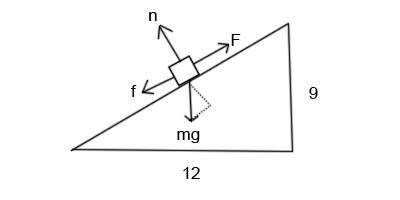Friction on an inclined plane

Homework Statement

Assume you are on a planet simillar to Earth where the acceleration due to gravity is 10 m/s2. A block of mass 15 kg lies on an incline plane. The height of the incline in 9m and the width is 12m. The coefficient of kinetic friction is .5. The magnitude of the force necessary to pull the block up the incline is most nearly:

114N, 290N, 344N, 428N, 86N, 244N, 150N, 184N, 108N, 122N

Homework Equations

$$\Sigma$$Fy=may

$$\Sigma$$Fx=max

fk=$$\mu$$kn

The Attempt at a Solution

Being that the block will be travelling at a constant speed, the sum of the forces in the x direction ought to equal zero. As for the y direction the sum of the forces must equal zero due to the normal forces and gravity cancelling eachother.

So for the forces in the y direction:

$$\Sigma$$Fy=n-mgcos$$\theta$$=0

The forces in the x direction:

$$\Sigma$$Fx=mgsin$$\theta$$-fk=0

$$\theta$$=tan-1(.75)=36.87

n=mgcos$$\theta$$ = 150(cos36.87)=119.999

fk=$$\mu$$kn=59.999

Which is clearly not close to any of the above options. I also tried using sin instead of cos incase I mixed up my directions but that yields me 90, which is close to one of the choices but not the correct answer. Thanks in advance.

Joe

rl.bhat
Homework Helper
Since the block is moving up, component of the weight along the inclined plane and frictional force act in the same direction.

So then my equation of motion for the x direction will become $$\Sigma$$Fx= mgsin$$\theta$$ + fk=0

Which means mgsin$$\theta$$=-fk

mgsin$$\theta$$=-$$\mu$$kn
n=180 which then gives me a value of 90 for fk which is the same answer.

What am I missing here? Thanks for the help.

Am I incorrect on my assumptions for the equations of motion, this is the only thing I can think of.

Joe

I also dont understand why the direction of the frictional force will be the same as the direction of motion. I thought that friction was by definition an opposing force which points opposite the direction of motion.

rock.freak667
Homework Helper
I also dont understand why the direction of the frictional force will be the same as the direction of motion. I thought that friction was by definition an opposing force which points opposite the direction of motion.

If you are using a force to move the mass up the incline, the frictional force will oppose this and hence act down the plane. The component of the weight parallel to the incline acts down the plane as well.

So you will have ∑Fx = F -mgsinθ-fk=0 which simplifies as

∑Fx = F -mgsinθ-μmgcosθ=0

so you can find F.

Also if you are given the opposite and adjacent lengths of the incline, usually they expect you to use the exact values of cosθ and sinθ so you don't have an error in rounding. I don't think it matters a lot here through.Where n is the reaction, f is the frictional force (μn), F is the force pulling the block, m is the mass of the block and g = 10 as stated

You can see that

mgcosθ = n

and

mgsinθ + f = F